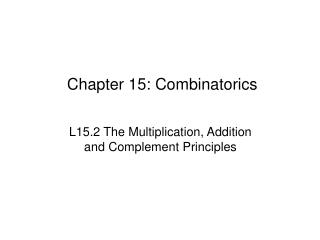Download PresentationChapter 15: Combinatorics

# Chapter 15: Combinatorics - PowerPoint PPT PresentationDownload Presentation## Chapter 15: Combinatorics

- - - - - - - - - - - - - - - - - - - - - - - - - - - E N D - - - - - - - - - - - - - - - - - - - - - - - - - - -
##### Presentation Transcript

1. Chapter 15: Combinatorics L15.2 The Multiplication, Addition and Complement Principles

2. Counting Principles Venn Diagrams together with the Inclusion – Exclusion Principle are useful. Other counting principles are used w/ more complex situations. Three principles are introduced in this section: • The Multiplication Principle • The Addition Principle • The Complement Principle

3. The Multiplication Principle: “and” If an action can be performed in n1 ways, and for each of these ways, another action can be performed in n2 ways, then the two actions can be performed together in n1·n2 ways. Example: Regular motor vehicle license plates in NJ consist of 3 letters, followed by 2 numbers, followed by another letter. Find the number of different license plates that can be generated: a) If there is no restriction on the letters or digits. b) If no two letters and no two digits can be repeated. 26 · 26 · 26 · 10 · 10 · 26 45,697,600 = = 26 · 25 · 24 · 10 · 9 · 23 32,292,000

4. , implies the product as Σ implies the sum. The Multiplication Principle Example: How many ways can six people line up in a line? · · · · · = 6 · 5 · 4 · 3 · 2 · 1 6! = 720 Factorial: n! = n(n – 1)(n – 2) ….. 3·2·1, n is a nonnegative integer Note: 0! = 1 Factorial on a Ti89: Within Math → Probability Menu or ◊ ÷ (hotkey)

5. The Addition Principle: “or” If two actions are mutually exclusive, and the first can be performed in n1 ways, and the second in n2 ways, then one action or the other can be done in n1 + n2 ways. Example: Mary can walk to work, but has other options. She owns 3 cars, 5 motorcycles, and 2 bicycles. How many ways can she get to work? + + + = 1 3 5 2 11 ways

6. The Multiplication & Addition Principles Multiplication is used when you select from set A and set B. Addition is used when you select from set A or set B. Example: If you have 3 shirts and 2 pairs of jeans, how many outfits can you make? 3 · 2 = 6 outfits Lets say you agree to loan a sibling either a shirt or a pair of jeans. How many different loans can be made? 3 + 2 = 5 loans

7. The Multiplication & Addition Principles Example: In Morse Code, the letters of the alphabet are represented by sequences of · and −. For example, · − represents ‘A’ and − − · represents ‘G’.Show that sequences of up to 4 symbols are needed to represent the entire alphabet. n1 = 2 Let n1 be the number of 1-symbol sequences. Let n2 be the number of 2-symbol sequences. Let n3 be the number of 3-symbol sequences. Let n4 be the number of 4-symbol sequences. n2 = 2·2 = 4 n3 = 2·2·2 = 8 n4 = 2·2·2·2 = 16 n1 + n2 + n3 + n4 = 30 Therefore a sequence of at least 4 symbols is needed to accommodate the 26-letter alphabet.

8. The Complement Principle If A is a subset of the set U, then n(A) = n(U) – n(A). Example: How many 3-digit numbers contain at least one 7? Let U = all 3-digit numbers Let A = 3-digit numbers containing at least one 7 Let A = 3 digit numbers containing no 7 n(A) = n(U) – n(A) 252 8 9 9 = · · – · · = 9 10 10 must exclude 0 must exclude 0 & 7 must exclude 7

9. Class Exercises Page 574 #1 – 8